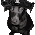## Monday, July 4, 2016

### Comparing Lease Terms

A common problem landlords face is deciding between competing lease offers between prospective tenants for a vacant space. For example, let's say a developer builds a 60k square foot office building in Silicon Valley. Let's assume the landlord leases the 30k square foot ground floor space and is now left with 30k square feet on the second floor. After a few months, the developer might have a few different offers from tenants for the vacant space. Each offer could have different terms. One offer might require more months of free rent, lower tenant improvement allowance, and a higher rent rate. Another might be a lower rent, but with less months of free rent. One tenant might have better credit which would lower the exit cap rate for the project. So how should a landlord decide which offer to run with?

Here's a link to a spreadsheet with an example of a lease comparison. The goal of the spreadsheet is to compare two lease offers with different terms by generating a single metric that will tell the landlord which lease is better for its project. The metric I decided to generate is an unlevered IRR. You might be confused on how it's possible to calculate an IRR for a lease, but when you think about it, a lease has all the ingredients needed to calculate an IRR, from the landlord's perspective. First, a lease transaction will usually have a large initial negative cash flow due to the landlord needing to pay a leasing commission and tenant improvement allowance (we'll also include the project cost per square foot multiplied by the suite square footage and add that to our initial cash outlay). Second, there's a stream of cash flows generated by the lease which is calculated by taking the rent payments minus the cost to operate the suite. Lastly, a lease will contribute to the overall value of a building when it's sold and we can estimate that contribution by taking the NOI the lease adds to the project and then dividing it by the exit cap rate. Once we calculate these three portions of the unlevered cash flows, we can then generate an IRR. The IRR can then be compared to the other lease terms and whichever has the higher IRR is probably the better deal.

The landlord's initial cash outlay includes a leasing commission, tenant improvement allowance, and we also include the suite's portion of the total project cost. Leasing commission can be tough to calculate. See this previous post to get a refresher on leasing commissions. I've included a somewhat automated way to calculate leasing commission in the spreadsheet. Just enter the lease terms for each lease and then create a leasing commission structure in the top right of the sheet. Once each leasing commission is calculated, we then need to calculate the tenant improvement allowance. Luckily this portion is much simpler and is just calculated as the suite square footage multiplied by the TI per square foot. Lastly, since we're factoring in the leases' contribution to the exit value of the project, we also need to factor in the how much it cost to purchase that suite, per se. That calculation is done by taking the overall project cost per square foot (not including TI's and LC's) and multiplying it by the suite size. We now have all three parts that make up the initial cash outlay for our IRR calculation.

The stream of cash flows generated by the lease is a fairly straightforward calculation, but there are a few wrinkles that need to be considered. The main assumptions for the calculation are the months of free rent, the initial rent rate, the yearly increase %, and the actual lease term. Take a look at the spreadsheet to see how the rent cash flow streams are calculated. The other part of it that needs to be calculated is the cost of operating the occupied suite. To factor it in, we take the stabilized project operating cost per square foot and multiply it by the suite square footage. We then need to net that opex out of the rent, giving us the lease's contribution to the project NOI for each period of our analysis.

Finally, we need to calculate the project's contribution to the exit value. This should be pretty simple and straightforward. We've already calculated the lease's contribution to NOI, so now we just take that NOI in our exit period and divide by the assumed project exit cap rate. There is one important wrinkle that should be noted. Different leases will affect the project's exit cap rate. A lease with an investment grade credit tenant, such as Apple, will lower the project's exit cap rate, so when comparing two leases, we need to adjust the exit cap rate for how the different leases might affect it.

Once you calculate the the unlevered cash flows for each lease, you can then calculate an IRR which will tell you which deal is more accretive to your project.

1.1.